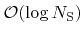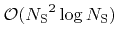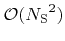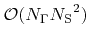Next: 5.1.2 Limitations Up: 5.1 Conventional Approach Previous: 5.1 Conventional Approach

## 5.1.1 Algorithmic Complexity

First the computational effort is considered, which is necessary for setting up the system matrix of (5.6). In principle, every pair of surface elements must be tested, if reemitted particles from one element can reach the other. A reasonable lower bound estimation for the complexity of such a visibility test is. Hence, the total complexity for the setup of the system matrix is at least.

Solving the system of linear equations also exhibits a bad scaling behavior. The expected number of non-zero entries is. A very efficient approach to solve the system is an iterative technique described in . In this way usually only a small number of matrix-vector multiplications is necessary to obtain a convergent solution. Since the complexity of a matrix-vector multiplication is, a quadratic scaling with surface size also governs the solution of the system.Next: 5.1.2 Limitations Up: 5.1 Conventional Approach Previous: 5.1 Conventional Approach

Otmar Ertl: Numerical Methods for Topography Simulation